# Computer Networking Assignment Help

11 de Feb de 2022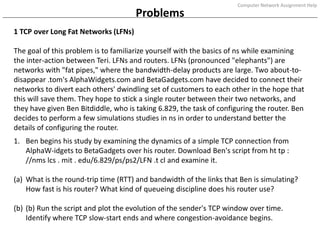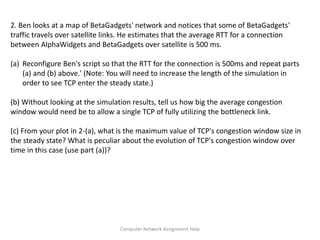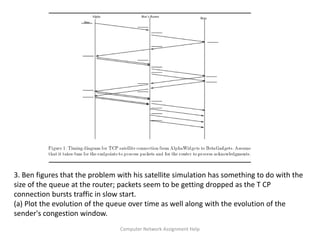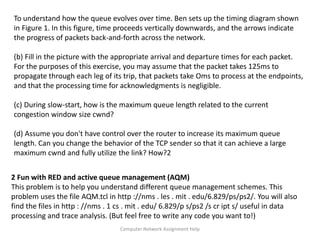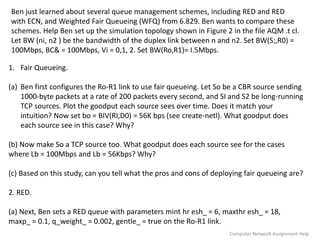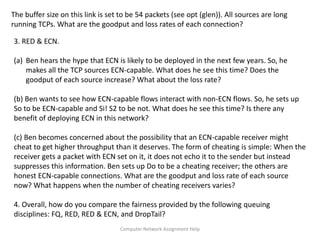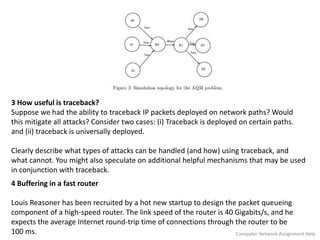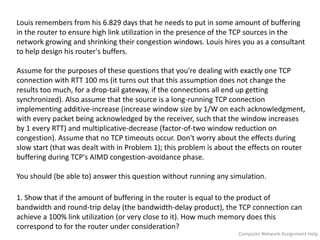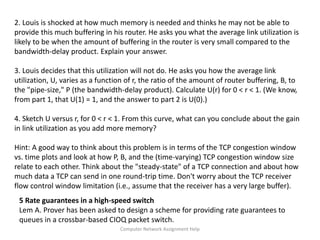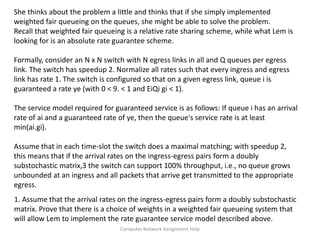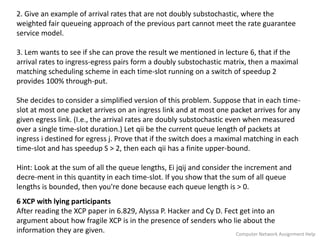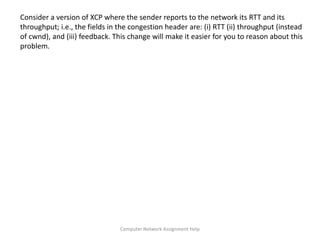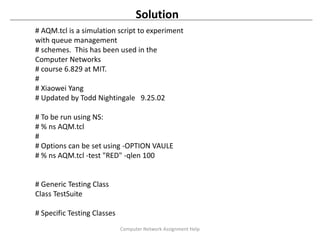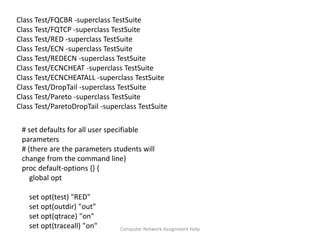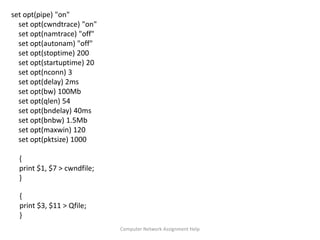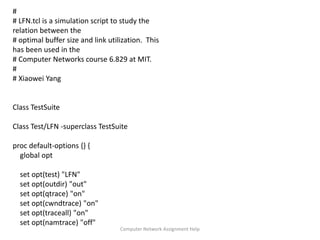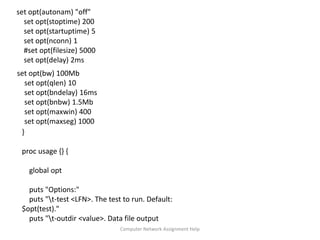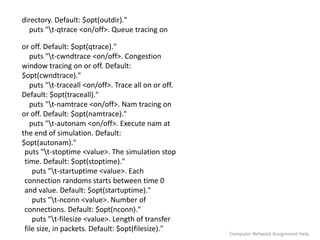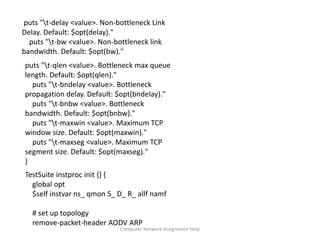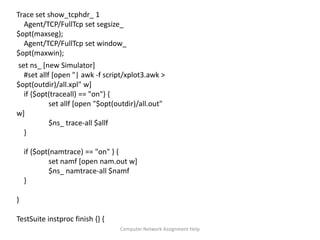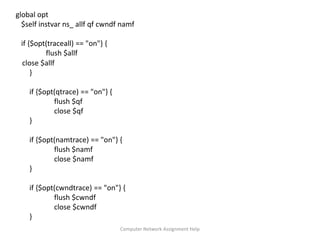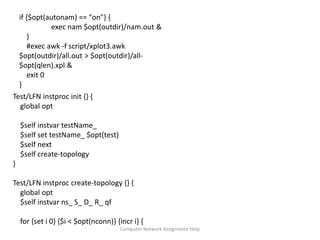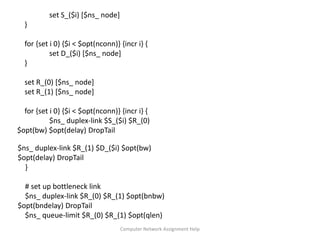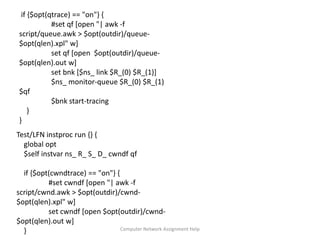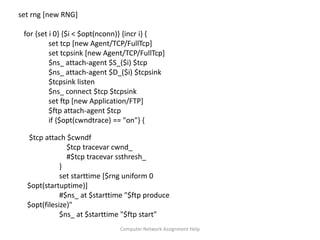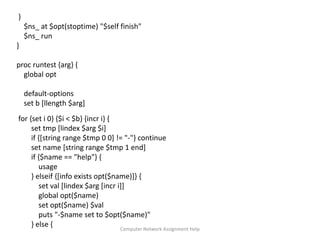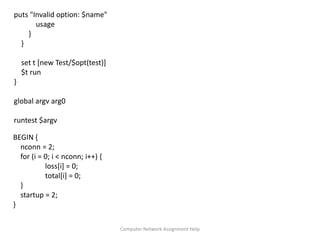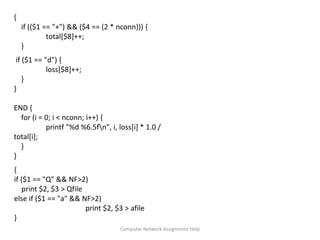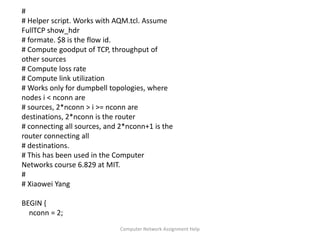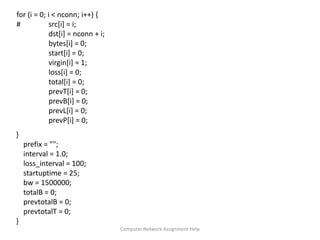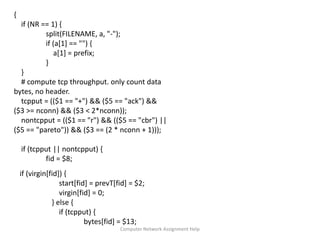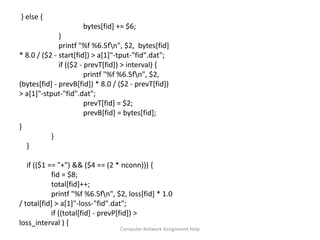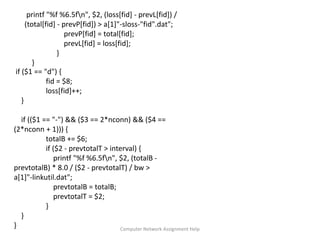1 de 34

### Computer Networking Assignment Help

• 1. For any help regarding Computer Network Assignment Help Visit :- https://www.computernetworkassignmenthelp.com/, Email :- support@computernetworkassignmenthelp.com or Call us at :- +1 678 648 4277
• 2. Problems 1 TCP over Long Fat Networks (LFNs) The goal of this problem is to familiarize yourself with the basics of ns while examining the inter-action between Teri. LFNs and routers. LFNs (pronounced "elephants") are networks with "fat pipes," where the bandwidth-delay products are large. Two about-to- disappear .tom's AlphaWidgets.com and BetaGadgets.com have decided to connect their networks to divert each others' dwindling set of customers to each other in the hope that this will save them. They hope to stick a single router between their two networks, and they have given Ben Bitdiddle, who is taking 6.829, the task of configuring the router. Ben decides to perform a few simulations studies in ns in order to understand better the details of configuring the router. 1. Ben begins his study by examining the dynamics of a simple TCP connection from AlphaW-idgets to BetaGadgets over his router. Download Ben's script from ht tp : //nms lcs . mit . edu/6.829/ps/ps2/LFN .t cl and examine it. (a) What is the round-trip time (RTT) and bandwidth of the links that Ben is simulating? How fast is his router? What kind of queueing discipline does his router use? (b) (b) Run the script and plot the evolution of the sender's TCP window over time. Identify where TCP slow-start ends and where congestion-avoidance begins. Computer Network Assignment Help
• 3. 2. Ben looks at a map of BetaGadgets' network and notices that some of BetaGadgets' traffic travels over satellite links. He estimates that the average RTT for a connection between AlphaWidgets and BetaGadgets over satellite is 500 ms. (a) Reconfigure Ben's script so that the RTT for the connection is 500ms and repeat parts (a) and (b) above.' (Note: You will need to increase the length of the simulation in order to see TCP enter the steady state.) (b) Without looking at the simulation results, tell us how big the average congestion window would need be to allow a single TCP of fully utilizing the bottleneck link. (c) From your plot in 2-(a), what is the maximum value of TCP's congestion window size in the steady state? What is peculiar about the evolution of TCP's congestion window over time in this case (use part (a))? Computer Network Assignment Help
• 4. 3. Ben figures that the problem with his satellite simulation has something to do with the size of the queue at the router; packets seem to be getting dropped as the T CP connection bursts traffic in slow start. (a) Plot the evolution of the queue over time as well along with the evolution of the sender's congestion window. Computer Network Assignment Help
• 5. To understand how the queue evolves over time. Ben sets up the timing diagram shown in Figure 1. In this figure, time proceeds vertically downwards, and the arrows indicate the progress of packets back-and-forth across the network. (b) Fill in the picture with the appropriate arrival and departure times for each packet. For the purposes of this exercise, you may assume that the packet takes 125ms to propagate through each leg of its trip, that packets take Oms to process at the endpoints, and that the processing time for acknowledgments is negligible. (c) During slow-start, how is the maximum queue length related to the current congestion window size cwnd? (d) Assume you don't have control over the router to increase its maximum queue length. Can you change the behavior of the TCP sender so that it can achieve a large maximum cwnd and fully utilize the link? How?2 2 Fun with RED and active queue management (AQM) This problem is to help you understand different queue management schemes. This problem uses the file AQM.tcl in http ://nms . les . mit . edu/6.829/ps/ps2/. You will also find the files in http : //nms . 1 cs . mit . edu/ 6.829/p s/ps2 /s cr ipt s/ useful in data processing and trace analysis. (But feel free to write any code you want to!) Computer Network Assignment Help
• 6. Ben just learned about several queue management schemes, including RED and RED with ECN, and Weighted Fair Queueing (WFQ) from 6.829. Ben wants to compare these schemes. Help Ben set up the simulation topology shown in Figure 2 in the file AQM .t cl. Let BW (ni, n2 ) be the bandwidth of the duplex link between n and n2. Set BW(S;,R0) = 100Mbps, BC& = 100Mbps, Vi = 0,1, 2. Set BW(Ro,R1)= I.5Mbps. 1. Fair Queueing. (a) Ben first configures the Ro-R1 link to use fair queueing. Let So be a CBR source sending 1000-byte packets at a rate of 200 packets every second, and SI and S2 be long-running TCP sources. Plot the goodput each source sees over time. Does it match your intuition? Now set bo = BIV(RI,D0) = 56K bps (see create-netl). What goodput does each source see in this case? Why? (b) Now make So a TCP source too. What goodput does each source see for the cases where Lb = 100Mbps and Lb = 56Kbps? Why? (c) Based on this study, can you tell what the pros and cons of deploying fair queueing are? 2. RED. (a) Next, Ben sets a RED queue with parameters mint hr esh_ = 6, maxthr esh_ = 18, maxp_ = 0.1, q_weight_ = 0.002, gentle_ = true on the Ro-R1 link. Computer Network Assignment Help
• 7. The buffer size on this link is set to be 54 packets (see opt (glen)). All sources are long running TCPs. What are the goodput and loss rates of each connection? 3. RED & ECN. (a) Ben hears the hype that ECN is likely to be deployed in the next few years. So, he makes all the TCP sources ECN-capable. What does he see this time? Does the goodput of each source increase? What about the loss rate? (b) Ben wants to see how ECN-capable flows interact with non-ECN flows. So, he sets up So to be ECN-capable and Si! S2 to be not. What does he see this time? Is there any benefit of deploying ECN in this network? (c) Ben becomes concerned about the possibility that an ECN-capable receiver might cheat to get higher throughput than it deserves. The form of cheating is simple: When the receiver gets a packet with ECN set on it, it does not echo it to the sender but instead suppresses this information. Ben sets up Do to be a cheating receiver; the others are honest ECN-capable connections. What are the goodput and loss rate of each source now? What happens when the number of cheating receivers varies? 4. Overall, how do you compare the fairness provided by the following queuing disciplines: FQ, RED, RED & ECN, and DropTail? Computer Network Assignment Help
• 8. 3 How useful is traceback? Suppose we had the ability to traceback IP packets deployed on network paths? Would this mitigate all attacks? Consider two cases: (i) Traceback is deployed on certain paths. and (ii) traceback is universally deployed. Clearly describe what types of attacks can be handled (and how) using traceback, and what cannot. You might also speculate on additional helpful mechanisms that may be used in conjunction with traceback. 4 Buffering in a fast router Louis Reasoner has been recruited by a hot new startup to design the packet queueing component of a high-speed router. The link speed of the router is 40 Gigabits/s, and he expects the average Internet round-trip time of connections through the router to be 100 ms. Computer Network Assignment Help
• 9. Louis remembers from his 6.829 days that he needs to put in some amount of buffering in the router to ensure high link utilization in the presence of the TCP sources in the network growing and shrinking their congestion windows. Louis hires you as a consultant to help design his router's buffers. Assume for the purposes of these questions that you're dealing with exactly one TCP connection with RTT 100 ms (it turns out that this assumption does not change the results too much, for a drop-tail gateway, if the connections all end up getting synchronized). Also assume that the source is a long-running TCP connection implementing additive-increase (increase window size by 1/W on each acknowledgment, with every packet being acknowledged by the receiver, such that the window increases by 1 every RTT) and multiplicative-decrease (factor-of-two window reduction on congestion). Assume that no TCP timeouts occur. Don't worry about the effects during slow start (that was dealt with in Problem 1); this problem is about the effects on router buffering during TCP's AIMD congestion-avoidance phase. You should (be able to) answer this question without running any simulation. 1. Show that if the amount of buffering in the router is equal to the product of bandwidth and round-trip delay (the bandwidth-delay product), the TCP connection can achieve a 100% link utilization (or very close to it). How much memory does this correspond to for the router under consideration? Computer Network Assignment Help
• 11. She thinks about the problem a little and thinks that if she simply implemented weighted fair queueing on the queues, she might be able to solve the problem. Recall that weighted fair queueing is a relative rate sharing scheme, while what Lem is looking for is an absolute rate guarantee scheme. Formally, consider an N x N switch with N egress links in all and Q queues per egress link. The switch has speedup 2. Normalize all rates such that every ingress and egress link has rate 1. The switch is configured so that on a given egress link, queue i is guaranteed a rate ye (with 0 < 9. < 1 and EiQi gi < 1). The service model required for guaranteed service is as follows: If queue i has an arrival rate of ai and a guaranteed rate of ye, then the queue's service rate is at least min(ai.gi). Assume that in each time-slot the switch does a maximal matching; with speedup 2, this means that if the arrival rates on the ingress-egress pairs form a doubly substochastic matrix,3 the switch can support 100% throughput, i.e., no queue grows unbounded at an ingress and all packets that arrive get transmitted to the appropriate egress. 1. Assume that the arrival rates on the ingress-egress pairs form a doubly substochastic matrix. Prove that there is a choice of weights in a weighted fair queueing system that will allow Lem to implement the rate guarantee service model described above. Computer Network Assignment Help
• 12. 2. Give an example of arrival rates that are not doubly substochastic, where the weighted fair queueing approach of the previous part cannot meet the rate guarantee service model. 3. Lem wants to see if she can prove the result we mentioned in lecture 6, that if the arrival rates to ingress-egress pairs form a doubly substochastic matrix, then a maximal matching scheduling scheme in each time-slot running on a switch of speedup 2 provides 100% through-put. She decides to consider a simplified version of this problem. Suppose that in each time- slot at most one packet arrives on an ingress link and at most one packet arrives for any given egress link. (I.e., the arrival rates are doubly substochastic even when measured over a single time-slot duration.) Let qii be the current queue length of packets at ingress i destined for egress j. Prove that if the switch does a maximal matching in each time-slot and has speedup S > 2, then each qii has a finite upper-bound. Hint: Look at the sum of all the queue lengths, Ei jqij and consider the increment and decre-ment in this quantity in each time-slot. If you show that the sum of all queue lengths is bounded, then you're done because each queue length is > 0. 6 XCP with lying participants After reading the XCP paper in 6.829, Alyssa P. Hacker and Cy D. Fect get into an argument about how fragile XCP is in the presence of senders who lie about the information they are given. Computer Network Assignment Help
• 13. Consider a version of XCP where the sender reports to the network its RTT and its throughput; i.e., the fields in the congestion header are: (i) RTT (ii) throughput (instead of cwnd), and (iii) feedback. This change will make it easier for you to reason about this problem. Computer Network Assignment Help
• 14. # AQM.tcl is a simulation script to experiment with queue management # schemes. This has been used in the Computer Networks # course 6.829 at MIT. # # Xiaowei Yang # Updated by Todd Nightingale 9.25.02 # To be run using NS: # % ns AQM.tcl # # Options can be set using -OPTION VAULE # % ns AQM.tcl -test "RED" -qlen 100 # Generic Testing Class Class TestSuite # Specific Testing Classes Solution Computer Network Assignment Help
• 15. Class Test/FQCBR -superclass TestSuite Class Test/FQTCP -superclass TestSuite Class Test/RED -superclass TestSuite Class Test/ECN -superclass TestSuite Class Test/REDECN -superclass TestSuite Class Test/ECNCHEAT -superclass TestSuite Class Test/ECNCHEATALL -superclass TestSuite Class Test/DropTail -superclass TestSuite Class Test/Pareto -superclass TestSuite Class Test/ParetoDropTail -superclass TestSuite # set defaults for all user specifiable parameters # (there are the parameters students will change from the command line) proc default-options {} { global opt set opt(test) "RED" set opt(outdir) "out" set opt(qtrace) "on" set opt(traceall) "on" Computer Network Assignment Help
• 16. set opt(pipe) "on" set opt(cwndtrace) "on" set opt(namtrace) "off" set opt(autonam) "off" set opt(stoptime) 200 set opt(startuptime) 20 set opt(nconn) 3 set opt(delay) 2ms set opt(bw) 100Mb set opt(qlen) 54 set opt(bndelay) 40ms set opt(bnbw) 1.5Mb set opt(maxwin) 120 set opt(pktsize) 1000 { print \$1, \$7 > cwndfile; } { print \$3, \$11 > Qfile; } Computer Network Assignment Help
• 17. # # LFN.tcl is a simulation script to study the relation between the # optimal buffer size and link utilization. This has been used in the # Computer Networks course 6.829 at MIT. # # Xiaowei Yang Class TestSuite Class Test/LFN -superclass TestSuite proc default-options {} { global opt set opt(test) "LFN" set opt(outdir) "out" set opt(qtrace) "on" set opt(cwndtrace) "on" set opt(traceall) "on" set opt(namtrace) "off" Computer Network Assignment Help
• 18. } proc usage {} { global opt puts "Options:" puts "t-test <LFN>. The test to run. Default: \$opt(test)." puts "t-outdir <value>. Data file output set opt(bw) 100Mb set opt(qlen) 10 set opt(bndelay) 16ms set opt(bnbw) 1.5Mb set opt(maxwin) 400 set opt(maxseg) 1000 set opt(autonam) "off" set opt(stoptime) 200 set opt(startuptime) 5 set opt(nconn) 1 #set opt(filesize) 5000 set opt(delay) 2ms Computer Network Assignment Help
• 19. or off. Default: \$opt(qtrace)." puts "t-cwndtrace <on/off>. Congestion window tracing on or off. Default: \$opt(cwndtrace)." puts "t-traceall <on/off>. Trace all on or off. Default: \$opt(traceall)." puts "t-namtrace <on/off>. Nam tracing on or off. Default: \$opt(namtrace)." puts "t-autonam <on/off>. Execute nam at the end of simulation. Default: \$opt(autonam)." directory. Default: \$opt(outdir)." puts "t-qtrace <on/off>. Queue tracing on puts "t-stoptime <value>. The simulation stop time. Default: \$opt(stoptime)." puts "t-startuptime <value>. Each connection randoms starts between time 0 and value. Default: \$opt(startuptime)." puts "t-nconn <value>. Number of connections. Default: \$opt(nconn)." puts "t-filesize <value>. Length of transfer file size, in packets. Default: \$opt(filesize)." Computer Network Assignment Help
• 20. puts "t-delay <value>. Non-bottleneck Link Delay. Default: \$opt(delay)." puts "t-bw <value>. Non-bottleneck link bandwidth. Default: \$opt(bw)." puts "t-qlen <value>. Bottleneck max queue length. Default: \$opt(qlen)." puts "t-bndelay <value>. Bottleneck propagation delay. Default: \$opt(bndelay)." puts "t-bnbw <value>. Bottleneck bandwidth. Default: \$opt(bnbw)." puts "t-maxwin <value>. Maximum TCP window size. Default: \$opt(maxwin)." puts "t-maxseg <value>. Maximum TCP segment size. Default: \$opt(maxseg)." } TestSuite instproc init {} { global opt \$self instvar ns_ qmon S_ D_ R_ allf namf # set up topology remove-packet-header AODV ARP Computer Network Assignment Help
• 21. Trace set show_tcphdr_ 1 Agent/TCP/FullTcp set segsize_ \$opt(maxseg); Agent/TCP/FullTcp set window_ \$opt(maxwin); set ns_ [new Simulator] #set allf [open "| awk -f script/xplot3.awk > \$opt(outdir)/all.xpl" w] if {\$opt(traceall) == "on"} { set allf [open "\$opt(outdir)/all.out" w] \$ns_ trace-all \$allf } if {\$opt(namtrace) == "on" } { set namf [open nam.out w] \$ns_ namtrace-all \$namf } } TestSuite instproc finish {} { Computer Network Assignment Help
• 22. global opt \$self instvar ns_ allf qf cwndf namf if {\$opt(traceall) == "on"} { flush \$allf close \$allf } if {\$opt(qtrace) == "on"} { flush \$qf close \$qf } if {\$opt(namtrace) == "on"} { flush \$namf close \$namf } if {\$opt(cwndtrace) == "on"} { flush \$cwndf close \$cwndf } Computer Network Assignment Help
• 23. if {\$opt(autonam) == "on"} { exec nam \$opt(outdir)/nam.out & } #exec awk -f script/xplot3.awk \$opt(outdir)/all.out > \$opt(outdir)/all- \$opt(qlen).xpl & exit 0 } Test/LFN instproc init {} { global opt \$self instvar testName_ \$self set testName_ \$opt(test) \$self next \$self create-topology } Test/LFN instproc create-topology {} { global opt \$self instvar ns_ S_ D_ R_ qf for {set i 0} {\$i < \$opt(nconn)} {incr i} { Computer Network Assignment Help
• 24. set S_(\$i) [\$ns_ node] } for {set i 0} {\$i < \$opt(nconn)} {incr i} { set D_(\$i) [\$ns_ node] } set R_(0) [\$ns_ node] set R_(1) [\$ns_ node] for {set i 0} {\$i < \$opt(nconn)} {incr i} { \$ns_ duplex-link \$S_(\$i) \$R_(0) \$opt(bw) \$opt(delay) DropTail \$ns_ duplex-link \$R_(1) \$D_(\$i) \$opt(bw) \$opt(delay) DropTail } # set up bottleneck link \$ns_ duplex-link \$R_(0) \$R_(1) \$opt(bnbw) \$opt(bndelay) DropTail \$ns_ queue-limit \$R_(0) \$R_(1) \$opt(qlen) Computer Network Assignment Help
• 25. if {\$opt(qtrace) == "on"} { #set qf [open "| awk -f script/queue.awk > \$opt(outdir)/queue- \$opt(qlen).xpl" w] set qf [open \$opt(outdir)/queue- \$opt(qlen).out w] set bnk [\$ns_ link \$R_(0) \$R_(1)] \$ns_ monitor-queue \$R_(0) \$R_(1) \$qf \$bnk start-tracing } } Test/LFN instproc run {} { global opt \$self instvar ns_ R_ S_ D_ cwndf qf if {\$opt(cwndtrace) == "on"} { #set cwndf [open "| awk -f script/cwnd.awk > \$opt(outdir)/cwnd- \$opt(qlen).xpl" w] set cwndf [open \$opt(outdir)/cwnd- \$opt(qlen).out w] } Computer Network Assignment Help
• 26. set rng [new RNG] for {set i 0} {\$i < \$opt(nconn)} {incr i} { set tcp [new Agent/TCP/FullTcp] set tcpsink [new Agent/TCP/FullTcp] \$ns_ attach-agent \$S_(\$i) \$tcp \$ns_ attach-agent \$D_(\$i) \$tcpsink \$tcpsink listen \$ns_ connect \$tcp \$tcpsink set ftp [new Application/FTP] \$ftp attach-agent \$tcp if {\$opt(cwndtrace) == "on"} { \$tcp attach \$cwndf \$tcp tracevar cwnd_ #\$tcp tracevar ssthresh_ } set starttime [\$rng uniform 0 \$opt(startuptime)] #\$ns_ at \$starttime "\$ftp produce \$opt(filesize)" \$ns_ at \$starttime "\$ftp start" Computer Network Assignment Help
• 27. } \$ns_ at \$opt(stoptime) "\$self finish" \$ns_ run } proc runtest {arg} { global opt default-options set b [llength \$arg] for {set i 0} {\$i < \$b} {incr i} { set tmp [lindex \$arg \$i] if {[string range \$tmp 0 0] != "-"} continue set name [string range \$tmp 1 end] if {\$name == "help"} { usage } elseif {[info exists opt(\$name)]} { set val [lindex \$arg [incr i]] global opt(\$name) set opt(\$name) \$val puts "-\$name set to \$opt(\$name)" } else { Computer Network Assignment Help
• 28. puts "Invalid option: \$name" usage } } set t [new Test/\$opt(test)] \$t run } global argv arg0 runtest \$argv BEGIN { nconn = 2; for (i = 0; i < nconn; i++) { loss[i] = 0; total[i] = 0; } startup = 2; } Computer Network Assignment Help
• 29. { if ((\$1 == "+") && (\$4 == (2 * nconn))) { total[\$8]++; } if (\$1 == "d") { loss[\$8]++; } } END { for (i = 0; i < nconn; i++) { printf "%d %6.5fn", i, loss[i] * 1.0 / total[i]; } } { if (\$1 == "Q" && NF>2) print \$2, \$3 > Qfile else if (\$1 == "a" && NF>2) print \$2, \$3 > afile } Computer Network Assignment Help
• 30. # # Helper script. Works with AQM.tcl. Assume FullTCP show_hdr # formate. \$8 is the flow id. # Compute goodput of TCP, throughput of other sources # Compute loss rate # Compute link utilization # Works only for dumpbell topologies, where nodes i < nconn are # sources, 2*nconn > i >= nconn are destinations, 2*nconn is the router # connecting all sources, and 2*nconn+1 is the router connecting all # destinations. # This has been used in the Computer Networks course 6.829 at MIT. # # Xiaowei Yang BEGIN { nconn = 2; Computer Network Assignment Help
• 31. for (i = 0; i < nconn; i++) { # src[i] = i; dst[i] = nconn + i; bytes[i] = 0; start[i] = 0; virgin[i] = 1; loss[i] = 0; total[i] = 0; prevT[i] = 0; prevB[i] = 0; prevL[i] = 0; prevP[i] = 0; } prefix = ""; interval = 1.0; loss_interval = 100; startuptime = 25; bw = 1500000; totalB = 0; prevtotalB = 0; prevtotalT = 0; } Computer Network Assignment Help
• 32. { if (NR == 1) { split(FILENAME, a, "-"); if (a == "") { a = prefix; } } # compute tcp throughput. only count data bytes, no header. tcpput = ((\$1 == "+") && (\$5 == "ack") && (\$3 >= nconn) && (\$3 < 2*nconn)); nontcpput = ((\$1 == "r") && ((\$5 == "cbr") || (\$5 == "pareto")) && (\$3 == (2 * nconn + 1))); if (tcpput || nontcpput) { fid = \$8; if (virgin[fid]) { start[fid] = prevT[fid] = \$2; virgin[fid] = 0; } else { if (tcpput) { bytes[fid] = \$13; Computer Network Assignment Help
• 33. } else { bytes[fid] += \$6; } printf "%f %6.5fn", \$2, bytes[fid] * 8.0 / (\$2 - start[fid]) > a"-tput-"fid".dat"; if ((\$2 - prevT[fid]) > interval) { printf "%f %6.5fn", \$2, (bytes[fid] - prevB[fid]) * 8.0 / (\$2 - prevT[fid]) > a"-stput-"fid".dat"; prevT[fid] = \$2; prevB[fid] = bytes[fid]; } } } if ((\$1 == "+") && (\$4 == (2 * nconn))) { fid = \$8; total[fid]++; printf "%f %6.5fn", \$2, loss[fid] * 1.0 / total[fid] > a"-loss-"fid".dat"; if ((total[fid] - prevP[fid]) > loss_interval ) { Computer Network Assignment Help
• 34. printf "%f %6.5fn", \$2, (loss[fid] - prevL[fid]) / (total[fid] - prevP[fid]) > a"-sloss-"fid".dat"; prevP[fid] = total[fid]; prevL[fid] = loss[fid]; } } if (\$1 == "d") { fid = \$8; loss[fid]++; } if ((\$1 == "-") && (\$3 == 2*nconn) && (\$4 == (2*nconn + 1))) { totalB += \$6; if (\$2 - prevtotalT > interval) { printf "%f %6.5fn", \$2, (totalB - prevtotalB) * 8.0 / (\$2 - prevtotalT) / bw > a"-linkutil.dat"; prevtotalB = totalB; prevtotalT = \$2; } } } Computer Network Assignment Help